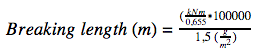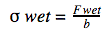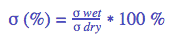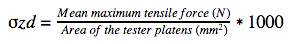Need help? Call us +31 20 40 99 300

## Strength properties

### Two-point bending stiffness (ISO 5628):

 What The force needed to bend the substrate without distorting its structure, i.e. without going beyond the elasticity modulus (E-modulus according to Young), expressed in mNm. Why Stiffness has impact on the runnability (trouble-free feeding through a press or printer), but also on the look and feel of the substrate. For some applications (folding board) it is essential, e.g. boxes, wishing cards etc. How In a stiffness meter a sample of defined dimensions is bent over an angle of 5° and a distance of 50 mm. Calculation Report the average force of 10 significant values in both MD and CD and the standard deviation.

### Two-point bending resistance (ISO 2493-1, TAPPI T556):

 What The force at the moment of bending the substrate can withstand without distorting its structure, i.e. without going beyond the elasticity modulus (E-modulus according to Young), expressed in mN. Why This is a property that has impact on converting operations for applications like wrapping paper, envelopes and some ways of bookbinding, but also on printing. How In a stiffness meter a sample of defined dimensions is bent over an angle of 15° or 7.5° and a distance of 50 or 10 mm. Calculation Report the average force of 10 significant values in both MD and CD and the standard deviation. Note This method only applies to paper up till 170 g/m².

### Tensile strength and – index (ISO 1924-2, TAPPI T494):

 What The maximum force (F-max) the substrate can withstand before it breaks, expressed in kN/m. Why On a press a lot of force is exerted on a substrate that may lead to deformation or rupture. How A strip of the substrate of 15 mm width is clamped between the grips of a tensile tester. Between the grips there is a distance of 180 mm. The upper grip travels with a speed of 25 ± 5 mm/min, until the substrate breaks. Calculation Report the average of 10 significant values in both MD and CD and the standard deviation. Note The tensile index is the average force divided by the weight of the substrate, expressed in N.m/g

### Stretch at break (ISO 1924-2, TAPPI T494):

 What The stretch of the fibre at the moment of break when the maximum force (F-max) is applied, expressed in %. Why The stretch of a fibre has impact on the forces a substrate may withstand. In MD a fibre has more strength but less stretch, in CD this is the other way around. How A strip of the substrate of 15 mm width is clamped between the grips of a tensile tester. Between the grips there is a distance of 180 mm. The upper grip travels with a speed of 25 ± 5 mm/min, until the substrate breaks. At precisely that moment the stretch is calculated. Calculation Report the average of 10 significant values in both MD and CD and the standard deviation.

### E-modulus according to Young (ISO 1924-2, TAPPI T494):

 What The extent of elasticity of the fibre, expressed in MPa. The E-modulus is a ratio calculated from tensile strength, stretch and stiffness of the sample. Why The stretch of a fibre has impact on the forces a substrate may withstand. As long as these forces are within the boundaries of the E-modulus, the substrate will keep its original structure. Due to greater speed of presses, thus generating more force, a printer needs to find a balance between speed and quality of the printed matter. How A strip of the substrate of 15 mm width is clamped between the grips of a tensile tester. Between the grips there is a distance of 180 mm. The upper grip travels with a speed of 25 ± 5 mm/min, until the substrate breaks. Calculation The E-modulus (E*) is calculated as:Where: ∆F = difference in force (N), ∆ li = difference in length (mm), li = initial length (mm) of sample, wi = width of sample, t = thickness of sample Report the average of 10 significant values in both MD and CD and the standard deviation.

### Tensile Energy Absorption (TEA) (ISO 1924-2, TAPPI T494):

 What The energy working equivalent, expressed in J/m², also known as the amount of energy necessary to break the sample. Why Any force exerted on the substrate generates energy; the amount of energy absorption is an indication of other strength properties: the more energy is absorbed; the more forces the substrate can withstand. How A strip of the substrate of 15 mm width is clamped between the grips of a tensile tester. Between the grips there is a distance of 180 mm. The upper grip travels with a speed of 25 ± 5 mm/min, until the substrate breaks. The TEA is calculated over the total duration of the test, till the moment of break. Calculation Report the average of 10 significant values in both MD and CD and the standard deviation.

## Breaking length (ISO 1924-2, TAPPI T494):

 What The theoretical length of a web with any width, that, when suspended freely, would break due to its own weight, expressed in metres. Why The theoretical length generates the tensile strength of the substrate. How A strip of the substrate of 15 mm width is clamped between the grips of a tensile tester. Between the grips there is a distance of 180 mm. The upper grip travels with a speed of 25 ± 5 mm/min, until the substrate breaks. CalculationReport the average of 10 significant values in both MD and CD and the standard deviation.

## Wet tensile strength and wet tensile index – Finch method (ISO 3781):

 What The maximum force (F-max) the substrate can withstand before it breaks, expressed in kN/m, after the sample has been immerged in distilled water during an agreed upon time, e.g. 1 hour. Why This has impact on wrapping material that may be exposed to moisture. How A strip of the substrate of 15 mm width is guided underneath a bar above a wet finch mounted on the lower grip of a tensile tester and both loose ends are clamped between the upper grip. Between the grip and the finch there is a distance of 90 mm. After immersion the upper grip travels with a speed of 25 ± 5 mm/min, until the substrate breaks. Calculationwhere: σ wet = wet tensile strength _ F wet = the mean maximum tensile force, in kilonewtons; b = the width of the dry test piece (15 mm), in metres.Report the average of 10 significant values in both MD and CD and the standard deviation. Note The wet tensile index is the average force divided by the weight of the substrate, expressed in N.m/g

### Wet tensile retention (ISO 3781):

 What Ratio between tensile strength wet and dry, expressed in a %. Why To determine loss of tensile strength after immersion in water. How Tensile strength (both dry and wet) is determined conforming to the appropriate ISO standards. CalculationWhere: σR = tensile strength retention σ wet = wet tensile strength, in kN/m σ dry = dry tensile strength, in kN/mReport the average of 10 significant values in both MD and CD and the standard deviation.

### Z-directional tensile strength/splitting resistance (ISO 15754, TAPPI T541):

 What Determination of the internal bond of the fibres, being the maximum force the surface of a substrate can withstand without splitting of layers and/or fibres, expressed in kPa. Why Because a substrate may not delaminate, blister or split due to ink tack. Tack value of ink is the biggest at the moment the printing cylinder releases from the substrate. How The surface of the substrate is clad with double sided tape at both sides, after which it is mounted between two metal blocks of an area of 645 mm² which are suspended between the grips of a tensile tester. First these blocks are compressed at a constant force during 6 seconds, after which the upper block is set into motion. At the moment the force diminishes due to splitting, the maximum force is reached. Calculationwhere: σ ZD = Z directional tensile strength_ F = mean maximum tensile force in N A = area of the tester platens in mm² (645 mm²)Report the average of at least 3 significant figures and the coefficient of variation. Note For papers having a weight less than 60 g/m², results shall be treated with caution since the tape may reinforce the paper.

### T-peel, resistance against pulling loose of self-adhesive material (EN-ISO 11339):

 What Determination of force needed to pull loose self-adhesive material that is adhered to itself, expressed in kN, thus determining the tack of the glue. Why Certain applications of self-adhesive material may need more tack than others (e.g. when the label needs to stick to a difficult surface like uncoated corrugated board) How The adhesive side facing each other, two samples of the same material of a width of preferably 25 mm are adhered together in such a way that the total length of the sample (≥ 200 mm) still has at least 50 mm left that does not adhere to each other. Before clamping the average thickness of the bond is determined. Both ends of 50 mm (X and Y in the figure above) are clamped in the grips of a tensile tester. The upper grip travels with a speed of 10 mm/min (or any other agreed upon speed) till at least 150 mm of the adhered end is pulled loose. Calculation Report the average force of at least 5 significant values over 100 mm, ignoring the first and last 25 mm of the peel, and the standard deviation.

### Tear and tear index according to Elmendorf (ISO 1974, TAPPI T414):

 What Resistance of a substrate against out of plane tearing, measured in average force and expressed in mN. Why Due to high speeds that can be gained on a modern press, forces may lead to tearing of the web. Also paper that complies with the Standards ISO 9706 and/or NEN 2728 pertaining to ageing resistance of paper needs to achieve a minimum force before and after artificial ageing. How A strip of the substrate with a height of 63 mm is clamped into the Elmendorf Pro Tear and pre-cut by a knife. Then a pendulum with a known weight is released, causing the substrate to tear. Generally, this test is performed with a layer of more sample strips at the same time. The amount of samples, gsm and thickness of one sheet are programmed beforehand so that software can determine the force for each single sample. Calculation Report the average of at least 10 significant values in both MD and CD and the standard deviation. Note The tear index is the average force divided by the average weight of the sample, expressed in mN.m²/g.

### Folding endurance according to Schopper method (ISO 5626, Tappi T423):

 What The number of times a substrate may be folded till it tears, expressed in log10 of the number of folds. Why For applications where the substrate needs to be often folded open and closed again, such as maps, installation drawings, building plans, it is important that the substrate does not tear at the crease. Also paper that complies with the Standards ISO 9706 and/or NEN 2728 pertaining to ageing resistance of paper, needs to achieve a minimum number of double folds before and after artificial ageing. How In a double fold tester strips of paper are clamped and mechanically folded two ways by a ‘knife’ with a constant force. A counter keeps track of the number of folds made. This number is converted to log10 values. Schematic view from above of one of the two positions on the Schopper apparatus: Test strip 100 x 15 mm Clamping length 90 mm Clamping force 8.68 N Folding clearance 10 mm off centre Speed 110 folds/min. Calculation Report the average of at least 10 significant values in both MD and CD and the standard deviation. Note Our tester can handle substrates with a maximum thickness of 225 µm (0.225 mm)